# Prove that ##AE=2BC## -Deductive Geometry

• chwala
My attempt on this...find my rough sketch here;In summary, from my analysis, the textbook has a mistake. I will keep trying tomorrow to make it work with different angles, but so far it does not comply with the identities.

#### chwala

Gold Member
Homework Statement
See attached
Relevant Equations
Geometry
This is the textbook question. I do not have the solution. I am pretty stuck on this one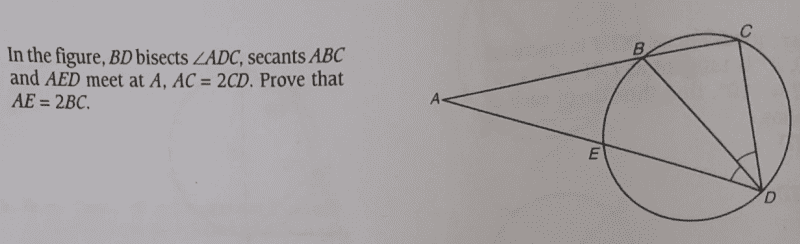My attempt on this...find my rough sketch here;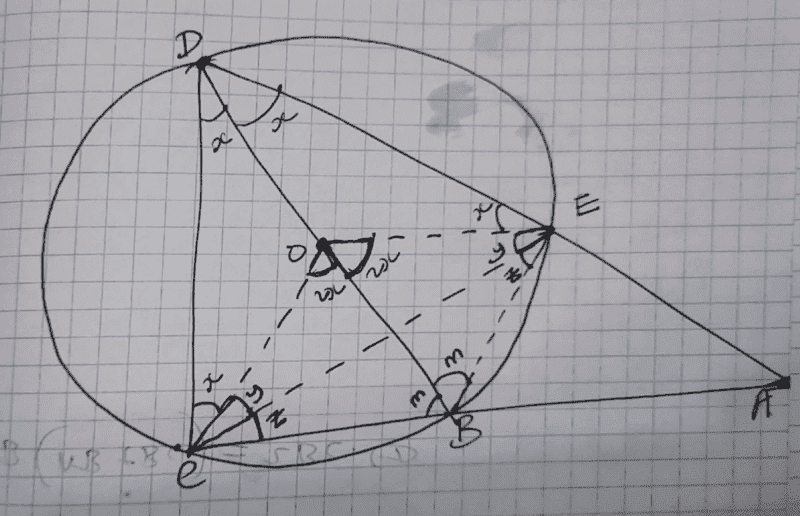From my analysis;
##x+x+m+m=180^0## angles opposite each other on a cyclic quadrilateral... I have point ##O## as the centre of the circle.

##x+y+z+x+y+z=180^0##

##⇒2(x+y+z)=180^0, x+y+z=90^0## therefore ##∠DEB= ∠AEB = 90^0##

Also i know that;

Last edited:
As
$$BC=BE$$
We should prove
$$\triangle DCA \sim \triangle BEA$$

•Lnewqban
I do not seem to get it...from my analysis;

Therefore; ##BD=2##units.

##∠ODC=∠ODE=30^0## each.

##∠(x+y+z)= 90^0, ∠y=∠z=30^0##each, ##∠m=60^0##

##∠OCBE## is a Rhombus of side ##1## unit.

##\sin 30^0= \dfrac{BC}{BD}##

##0.5= \dfrac{BC}{2}⇒BC=1##unit.

##∠AEB= 90^0##

##∠BAE=30^0## therefore ##\sin 30^0= \dfrac{BE}{AB}##

##0.5= \dfrac{1}{AB}⇒AB=2##unit.

We know from pythagoras theorem that ##AE^2+BE^2=AB^2##

##AE=\sqrt{3}## which does not conform to what we want. Am i missing something here?

Last edited:
...Maybe it ought to be ##AB= 2BC##

Last edited:
anuttarasammyak said:
As
$$BC=BE$$
We should prove
$$\triangle DCA \sim \triangle BEA$$
We see
$$\angle ABE=\angle CDE$$
by drawing OE,OB and OC where O is center of the circle, which is not on BD,with attention to
$$\angle BOE = \angle BOC = 2 \angle BDE$$
$$OB=OE=OC$$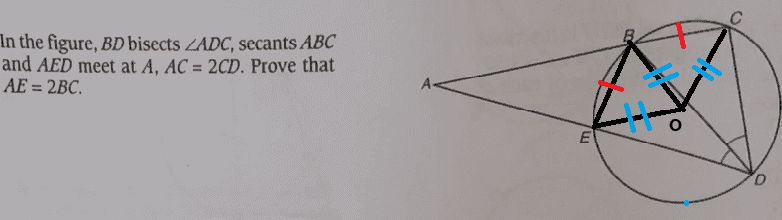Last edited:
•chwala
anuttarasammyak said:
We see
$$\angle ABE=\angle CDE$$
by drawing OE,OB and OC where O is center of the circle, which is not on BD.
I have no dispute on this...my working has factored in all that...

Last edited:
anuttarasammyak said:
We see
$$\angle ABE=\angle CDE$$
by drawing OE,OB and OC where O is center of the circle, which is not on BD,with attention to
$$\angle BOE = \angle BOC = 2 \angle BDE$$
$$OB=OE=OC$$View attachment 315334
Are you of the opinion that ##AE= 2BC##? As is indicated?

Yes. More in general
$$\frac{AC}{CD}=\frac{AE}{EB}$$
for not only 2 but any ratio value.

Last edited: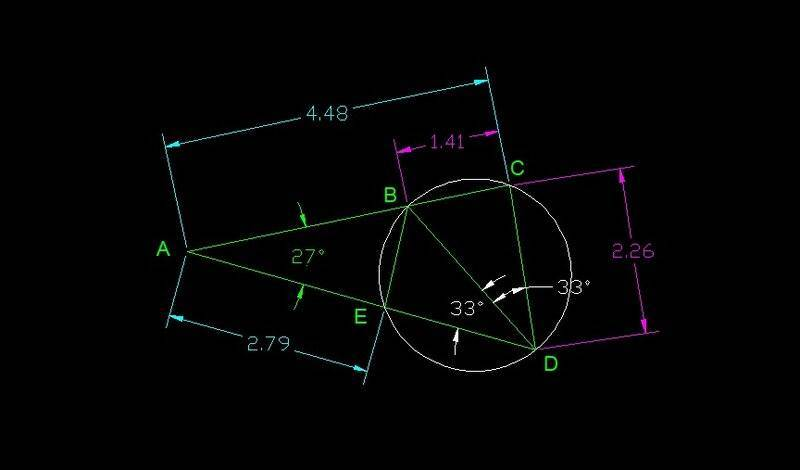•chwala
Lnewqban said:
Phew man! How were you able to determine the angles?

chwala said:
Phew man! How were you able to determine the angles?
I have been trying to make it work with different angles, but so far it does not comply with the identities.
I will keep trying tomorrow.

•chwala
Lnewqban said:
I have been trying to make it work with different angles, but so far it does not comply with the identities.
I will keep trying tomorrow.
Cheers @Lnewqban ...smart!

•Lnewqban
Lnewqban said:
...still a problem there...##AE≠2BC##

Last edited:
chwala said:
...still a problem there...##AE## is NOT twice ##BC##
Exactly!
That is why more work is needed.
If angle ACD is a 90, then angle EAB must be the arctan of 0.5 (as AC=2CD).
However, that does not work either.

•chwala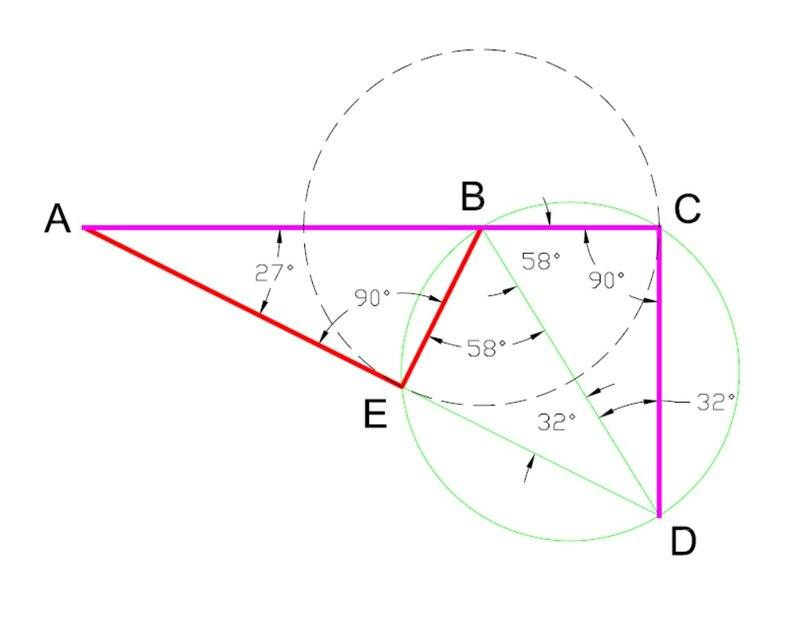Looking at it again...in my opinion i tend to think the concept being tested on this question is congruency. In answering this question it is paramount to use a given circle property.

Using the original diagram as shown in post ##1## for our understanding/view, I may state as follows;

##ΔABE## is congruent to triangle ##ΔDBE##.

##∠BEA=∠BED=90^0##.

(Refer to my post ##3## for the calculations on the numerical values given below)

Considering line ##BE## as the line of symmetry and knowing that ##BC=BE=1## unit then

##⇒AB=DB=2##units and

##⇒AE=DE=\sqrt{3}##units.

Clearly, the textbook has a mistake! The correct statement should have been

Prove ##AB=2BC##.

Last edited:
Lnewqban said:
I take it as an approximation because tan 64 degrees = 2.05030384158... ##\neq## 2.

[EDIT] We should take it arctan 2 for the exact solution.

chwala said:
Clearly, the textbook has a mistake!
I would like to know how you feel skeptic on my post #5.

Last edited:
anuttarasammyak said:
We see
$$\angle ABE=\angle CDE$$
by drawing OE,OB and OC where O is center of the circle, which is not on BD,with attention to
$$\angle BOE = \angle BOC = 2 \angle BDE$$
$$OB=OE=OC$$View attachment 315334
According to me the centre of the circle i.e point ##O## is on the line ##BD## but according to you it is not. Of course, i could be wrong!

Last edited:
Some thoughts on a way to measure the lengths in general configuration.

O: unit circle with B,E,C.D on it. In cartesian coordinates
##B(1,0),E(cos \theta, sin\theta),C(cos\theta,-sin\theta),D(cos\phi,sin\phi)##

Equation of line BC: ##y=\frac{sin\theta}{1-\cos\theta}(x-1)##

Equation of lne DE: ## y-\sin\theta = \frac{\sin\theta-\sin\phi}{\cos\theta-\cos\phi}(x-\sin\theta)##
Thus
$$A(\frac{\sin\theta(1-\frac{\sin\theta-\sin\phi}{\cos\theta-\cos\phi})+\frac{\sin\theta}{1-\cos\theta}}{\frac{sin\theta}{1-\cos\theta}-\frac{\sin\theta-\sin\phi}{\cos\theta-\cos\phi}}, \frac{\sin\theta}{1-\cos\theta} \frac{\sin\theta(1-\frac{\sin\theta-\sin\phi}{\cos\theta-\cos\phi})+\frac{\sin\theta}{1-\cos\theta}}{\frac{sin\theta}{1-\cos\theta}-\frac{\sin\theta-\sin\phi}{\cos\theta-\cos\phi}} - \frac{\sin\theta}{1-\cos\theta} )$$

By them we can get formula of side length of triangles in general configuration.

chwala said:
According to me the centre of the circle i.e point O is on the line BD but according to you it is not. Of course i could be wrong.
"O is on BD or not" and "the ration of 2" have nothing to do with my proof. That may be so for the ratio "2", may be not, and may be so for the ratio "3", may be not and so on.

anuttarasammyak said:
We see
$$\angle ABE=\angle CDE$$
by drawing OE,OB and OC where O is center of the circle, which is not on BD,with attention to
$$\angle BOE = \angle BOC = 2 \angle BDE$$
$$OB=OE=OC$$View attachment 315334
I was reacting to the highlighted and not your proof.

anuttarasammyak said:
"O is on BD or not" and "the ration of 2" have nothing to do with my proof. That may be so for the ratio "2", may be not, and may be so for the ratio "3", may be not and so on.
Noted man. CheersSo did you find that O is on BD in the condition of the problem ? I am intereseted in such a coincidence for me.

Last edited:
•chwala
anuttarasammyak said:
So did you find that O is on BD in the setting of the problem ?
Hi @anuttarasammyak. I do not seem to get you...the question is as it is from the textbook...i am looking at the problem just the same way you're looking at it.

I am not sure if point ##O## i.e the centre of the circle lies on line ##BD##.

In such questions like i indicated earlier in my post ##16##, one has to apply the properties of the circle in answering the question. That is the start point i guess...that could be a herculian task if we go with your approach of say, taking point ##O## not lying on the line ##BD##. With my approach, it could be straightforward as we are dealing with standard angles as shown in my previous post ##3## and ##16##.

Last edited:
O is center point of the circle.

[EDIT] Now I come to an idea that we can choose the configuration of diameter BD by adjusting circumference angles if we may in order to satisfy the ration of the sides given.

Last edited:
anuttarasammyak said:
I take it as an approximation because tan 64 degrees = 2.05030384158... ##\neq## 2.
...
It is only the resolution with which the software represents the angles; I could increase it later to show that the angle's values are exactly what should be to satisfy the 1:2 proportion of the sides of the triangles.

That last drawing shows two 1:2 proportion sides in red for one triangle and two sides in magenta for the other triangle.

Rather than starting with angles, I followed the description of the problem: two secants and one bisector.
Then, I draw the green circle using three points of reference (B, C and D), not knowing where the center would located at.
The green and the dashed circles both pass by point E naturally.

The only liberty I took was assuming the 90 degree angle.
For any combination, the bisector passes by the center of the circle, which makes the top lines symmetrical (note dashed line circle).

Lnewqban said:
It is only the resolution with which the software represents the angles; I could increase it later to show that the angle's values are exactly what should be to satisfy the 1:2 proportion of the sides of the triangles.

That last drawing shows two 1:2 proportion sides in red for one triangle and two sides in magenta for the other triangle.

Rather than starting with angles, I followed the description of the problem: two secants and one bisector.
Then, I draw the green circle using three points of reference (B, C and D), not knowing where the center would located at.
The green and the dashed circles both pass by point E naturally.

The only liberty I took was assuming the 90 degree angle.
For any combination, the bisector passes by the center of the circle, which makes the top lines symmetrical (note dashed line circle).
You may have a valid point...but remember this is a textbook question that may not warrant use of software in a class setting. In other words, how would students show the proof as required?

Lnewqban said:
Rather than starting with angles, I followed the description of the problem: two secants and one bisector.
Then, I draw the green circle using three points of reference (B, C and D), not knowing where the center would located at.
The green and the dashed circles both pass by point E naturally.

The only liberty I took was assuming the 90 degree angle.
Thanks for the explanation. I am not sure and interested in whether we can always choose 90 degree angle configuration, i.e. BD is diameter of the circle, for not only 2 but any given ratio of the sides by adjusting circumference angles. It may be.

chwala said:
You may have a valid point...but remember this is a textbook question that may not warrant use of software in a class setting. In other words, how would students show the proof as required?
I don't know how to prove it, reason for which I have used a drawing at scale.
This time, I started from a 2-unit horizontal line followed by a 1-unit vertical line (ACD magenta lines), and ended up with a 1:2 proportion red lines.
Therefore, at least two of the formed triangles must be proprtional (1:2 sides plus one similar angle).

•chwala
@anuttarasammyak , attached is the same drawing, with improved resolution for the angles and measurements of the sides of the triangles.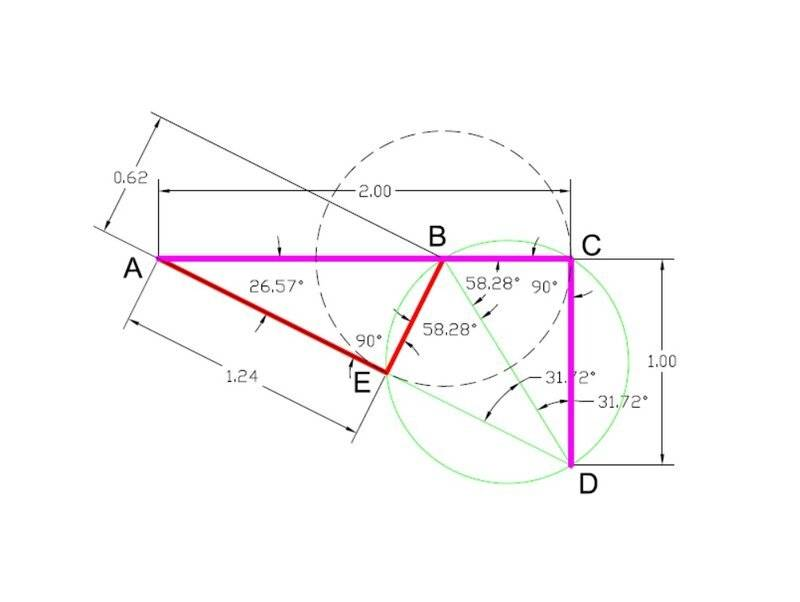•anuttarasammyak
Lnewqban said:
@anuttarasammyak , attached is the same drawing, with improved resolution for the angles and measurements of the sides of the triangles.
View attachment 315404
Nice ...what's your ultimate goal/intention on having different drawings on the problem? are the measurements of ##AE## and## BC## tending towards the envisaged value? Or drifting away...cheers.

Last edited:
chwala said:
Looking at it again...in my opinion i tend to think the concept being tested on this question is congruency. In answering this question it is paramount to use a given circle property.

. . .

Clearly, the textbook has a mistake! The correct statement should have been

Prove ##AB=2BC##.
I agree with @anuttarasammyak .

The textbook problem is solvable.

As he said, you simply need to show that ##\displaystyle \triangle DCA \sim \triangle BEA## . From this you get that ##AE=2EB## .

Also, point ##O## is not necessarily on ##BD## .

Last edited:
•anuttarasammyak
SammyS said:
I agree with @anuttarasammyak .

The textbook problem is solvable.

As he said, you simply need to show that ##\displaystyle \triangle DCA \sim \triangle BEA## . From this you get that ##AE=2EB## .

Also, point ##O## is not necessarily on ##BD## .
Kindly find my rejoinder...sorry am a bit busy had to scribble my response.
I have made use of similarity (on the diagram) as is suggested...and I still cannot see how ##BC= EB##.

#### Attachments

Last edited:There is no mention in the problem that ##\angle ACD## is rectangle or BD is diameter. BD can be a diameter as @Lnewqban showed. But it is not a requirement coming from AC = 2CD that BD is a diameter.

Last edited:
•nasu
anuttarasammyak said:
View attachment 315462
There is no mention in the problem that ##\angle ACD## is rectangle or BD is diameter. AC = 2CD does not require that BD is a diameter.
If indeed it's not a diameter then we cannot talk of Similarity. That would mean that we are looking at the other option of Congruency. In that case, it would be interesting to see which postulate applies.

•anuttarasammyak